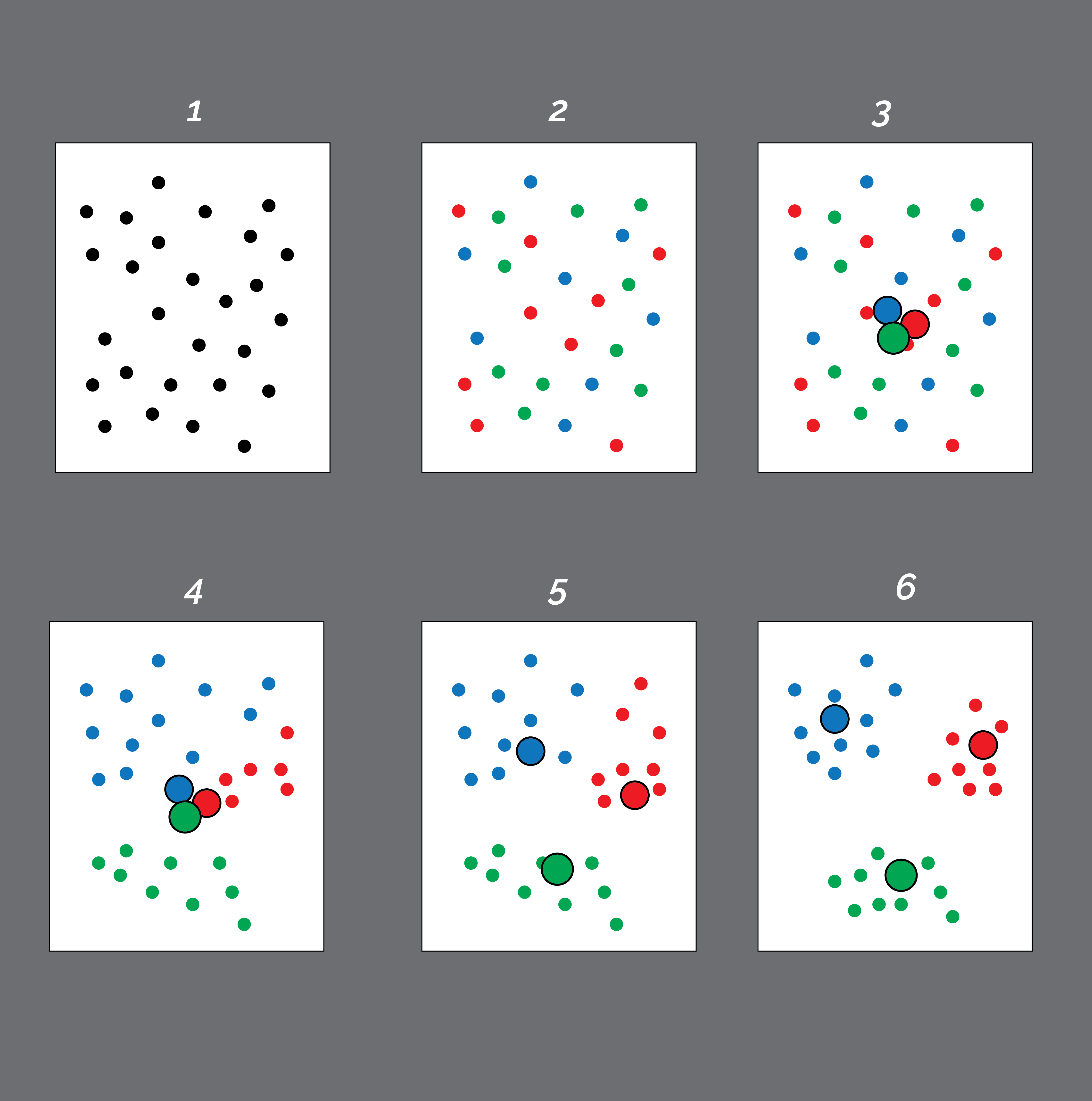a shot of dev knowledge

RELATED TAGS

What is the K-means algorithm?

What is the K-means algorithm?

Imagine an algorithm that is very fast and efficient in categorizing data points into sub-groups with little information. The whole reason for classification tasks in unsupervised machine learning is to correctly distinguish which data points belong to which group, and K-Means Clustering Algorithm does just that.

Clustering is a method used in exploratory data analysis to unravel similarities and insights about the structure of the data. It can be said to be the method for pinpointing where data points can be categorized into smaller groups in the data. The smaller groups, known as clusters, have data points that are alike in specific respects, while data points that belong to different clusters are dissimilar.

Clustering is considered an unsupervised learning method because the target classes aren’t provided in order to compare the output of the clusters against, to evaluate its performance. This algorithm can be used to monitor students’ academic performance, for example. Based on the students’ score, they would be grouped into different clusters.

Clustering can also be used in market segmentation, where the clusters are created based on the spending or behavioral attributes of customers. Among other uses, clustering can be using for drug activity predictions, spam filtering, identifying fake news, etc.

The k-means algorithm is an iterative algorithm that tries to partition the datasets into K pre-defined, distinct, non-overlapping subgroups (clusters), where each data point belongs to only one group.

The working of the algorithm:

#K Means cluster creation
from sklearn import KMeans
#creating an instance of a K Means model with 3 clusters
Kmeans=KMeans(n_Clusters=2)
kmeans.fit(x)
Kmeans.cluster_centers_

• Choose a number of clusters ‘K’.

• Randomly assign each data point to a cluster until the clusters stop adjusting.

Repeat the following steps:

• Compute the cluster centroid by taking the mean vector of points in the cluster.

• Assign each data point to the cluster for which the centroid is the closest.

The approach that K-means follows to solve the problem is called Expectation-Maximization.

• The E-step is assigning the data points to the closest cluster.

• The M-step is computing the centroid of each cluster.• The box labelled ‘1’ is your plane data – the observations.

• Each data point is then randomly assigned to a cluster, as seen in the second box.

• The box on the top-right, labelled ‘3’, is the stage where the cluster centroids are computed. The centroids (the larger circles) are not distinctly placed because the initial cluster assignment for the data points were chosen at random.

• In the fourth box, each observation is assigned to the nearest centroid.

• The centroid is then readjusted and step 3 is repeated.

• These steps are done over again until there are no new centroids, as seen in the last box.

What is the Elbow method?

When choosing a K-value, it is important to remember that there is no best way to choose a K-value. Choosing this value comes with experience and domain knowledge. Regardless, there are a number of ways this can be done, one of these is the ‘Elbow method.’

The Elbow method gives us an idea on a k number of clusters based on the sum of squared distance (SSE) between data points and their assigned clusters’ centroids. We pick k at the spot where SSE decreases abruptly. This produces an ‘elbow effect’ on the graph.

In conclusion: K-Means clustering algorithm is a good algorithm mostly used by machine learning engineers when dealing with clustering problems. It does a decent job in blindly recognizing patterns and effectively classifying data points.

References

RELATED TAGS

RELATED COURSES

View all Courses

Keep Exploring

Learn in-demand tech skills in half the time